# 3 DIFFERENTIATION RULES DIFFERENTIATION RULES 3 4 The

• Slides: 57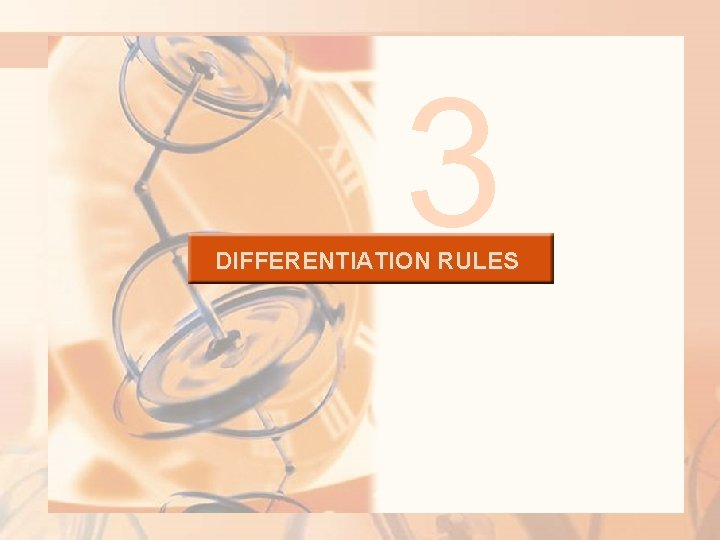3 DIFFERENTIATION RULESDIFFERENTIATION RULES 3. 4 The Chain Rule In this section, we will learn about: Differentiating composite functions using the Chain Rule.CHAIN RULE Suppose you are asked to differentiate the function § The differentiation formulas you learned in the previous sections of this chapter do not enable you to calculate F’(x).CHAIN RULE Observe that F is a composite function. In fact, if we let and let u = g(x) = x 2 + 1, then we can write y = F(x) = f (g(x)). That is, F = f ◦ g.CHAIN RULE We know how to differentiate both f and g. So, it would be useful to have a rule that shows us how to find the derivative of F = f ◦ g in terms of the derivatives of f and g.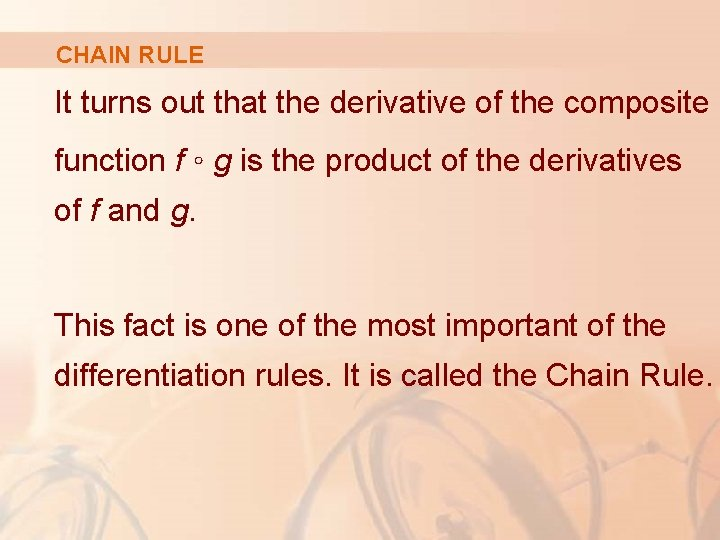CHAIN RULE It turns out that the derivative of the composite function f ◦ g is the product of the derivatives of f and g. This fact is one of the most important of the differentiation rules. It is called the Chain Rule.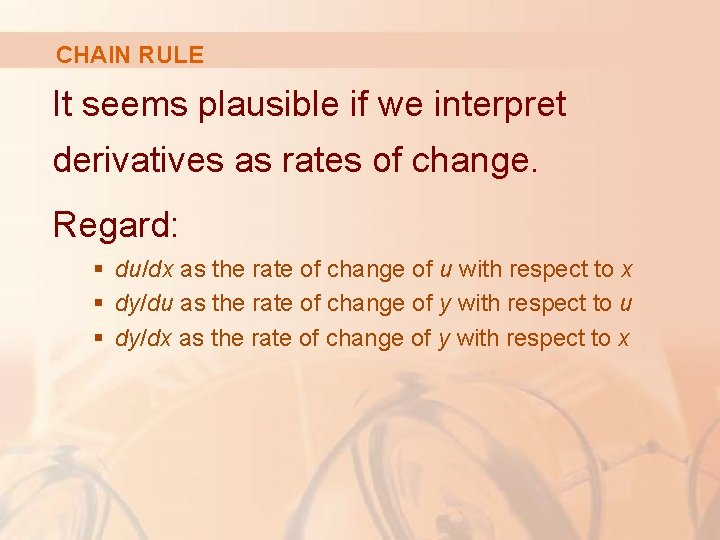CHAIN RULE It seems plausible if we interpret derivatives as rates of change. Regard: § du/dx as the rate of change of u with respect to x § dy/du as the rate of change of y with respect to u § dy/dx as the rate of change of y with respect to x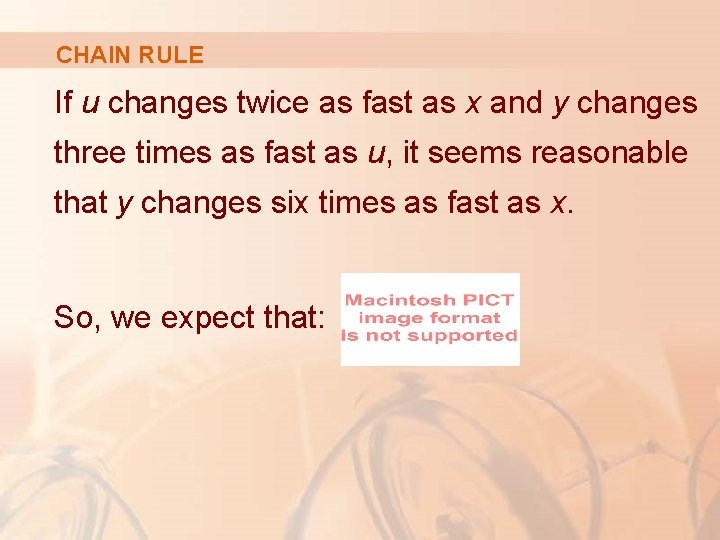CHAIN RULE If u changes twice as fast as x and y changes three times as fast as u, it seems reasonable that y changes six times as fast as x. So, we expect that:THE CHAIN RULE If g is differentiable at x and f is differentiable at g(x), the composite function F = f ◦ g defined by F(x) = f(g(x)) is differentiable at x and F’ is given by the product: F’(x) = f’(g(x)) • g’(x) § In Leibniz notation, if y = f(u) and u = g(x) are both differentiable functions, then: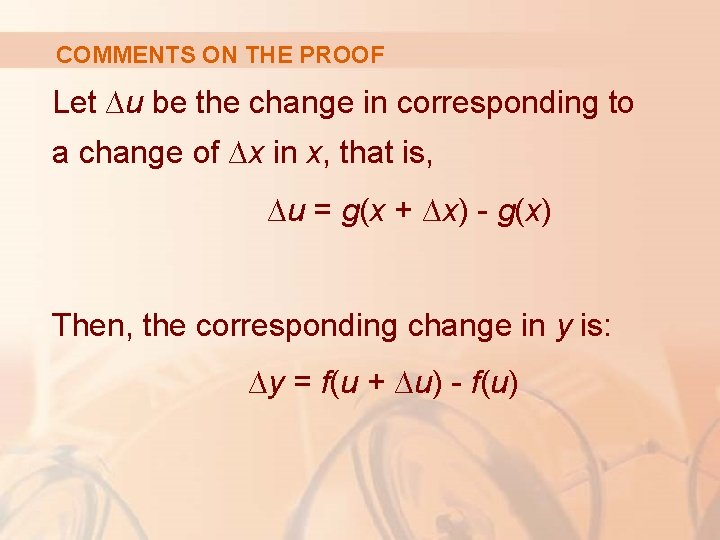COMMENTS ON THE PROOF Let ∆u be the change in corresponding to a change of ∆x in x, that is, ∆u = g(x + ∆x) - g(x) Then, the corresponding change in y is: ∆y = f(u + ∆u) - f(u)COMMENTS ON THE PROOF It is tempting to write: Equation 1COMMENTS ON THE PROOF The only flaw in this reasoning is that, in Equation 1, it might happen that ∆u = 0 (even when ∆x ≠ 0) and, of course, we can’t divide by 0.COMMENTS ON THE PROOF Nonetheless, this reasoning does at least suggest that the Chain Rule is true. § A full proof of the Chain Rule is given at the end of the section.CHAIN RULE Equations 2 and 3 The Chain Rule can be written either in the prime notation (f ◦ g)’(x) = f’(g(x)) • g’(x) or, if y = f(u) and u = g(x), in Leibniz notation:CHAIN RULE Equation 3 is easy to remember because, if dy/du and du/dx were quotients, then we could cancel du. However, remember: § du has not been defined § du/dx should not be thought of as an actual quotientCHAIN RULE E. g. 1—Solution 1 Find F’(x) if § One way of solving this is by using Equation 2. § At the beginning of this section, we expressed F as F(x) = (f ◦ g))(x) = f(g(x)) where and g(x) = x 2 + 1.CHAIN RULE § Since we have E. g. 1—Solution 1CHAIN RULE E. g. 1—Solution 2 We can also solve by using Equation 3. § If we let u = x 2 + 1 and then: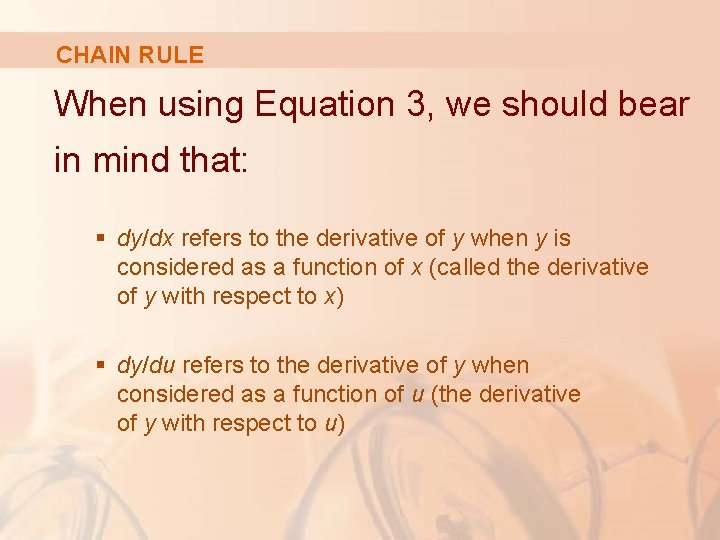CHAIN RULE When using Equation 3, we should bear in mind that: § dy/dx refers to the derivative of y when y is considered as a function of x (called the derivative of y with respect to x) § dy/du refers to the derivative of y when considered as a function of u (the derivative of y with respect to u)CHAIN RULE For instance, in Example 1, y can be considered as a function of x and also as a function of u § Note that: .NOTE In using the Chain Rule, we work from the outside to the inside. § Equation 2 states that we differentiate the outer function f [at the inner function g(x)] and then we multiply by the derivative of the inner function.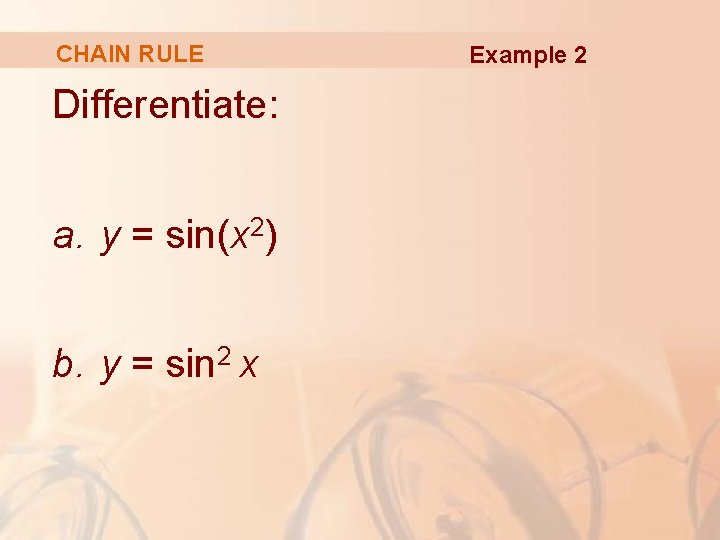CHAIN RULE Differentiate: a. y = sin(x 2) b. y = sin 2 x Example 2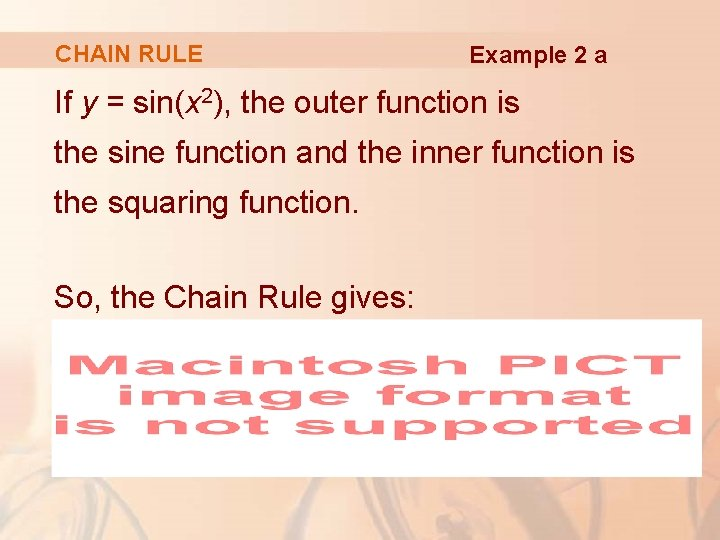CHAIN RULE Example 2 a If y = sin(x 2), the outer function is the sine function and the inner function is the squaring function. So, the Chain Rule gives: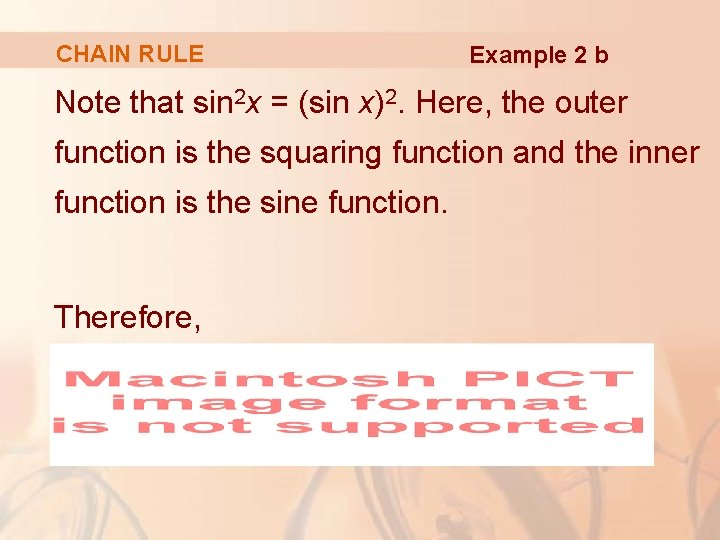CHAIN RULE Example 2 b Note that sin 2 x = (sin x)2. Here, the outer function is the squaring function and the inner function is the sine function. Therefore,CHAIN RULE Example 2 b The answer can be left as 2 sin x cos x or written as sin 2 x (by a trigonometric identity known as the double-angle formula).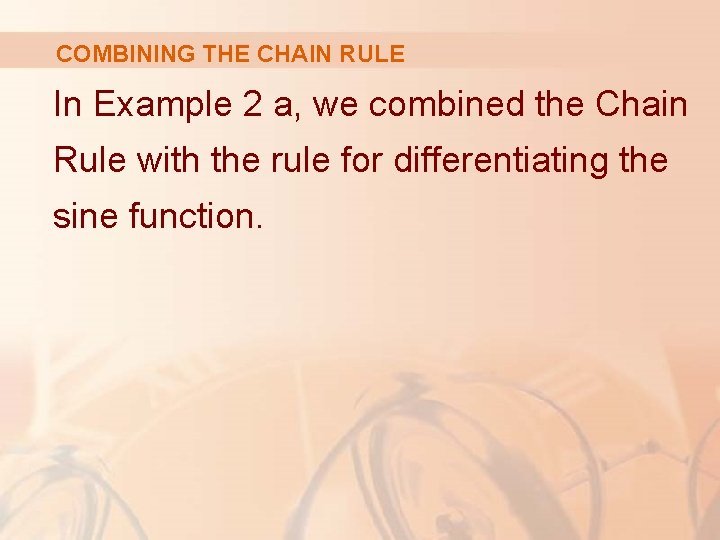COMBINING THE CHAIN RULE In Example 2 a, we combined the Chain Rule with the rule for differentiating the sine function.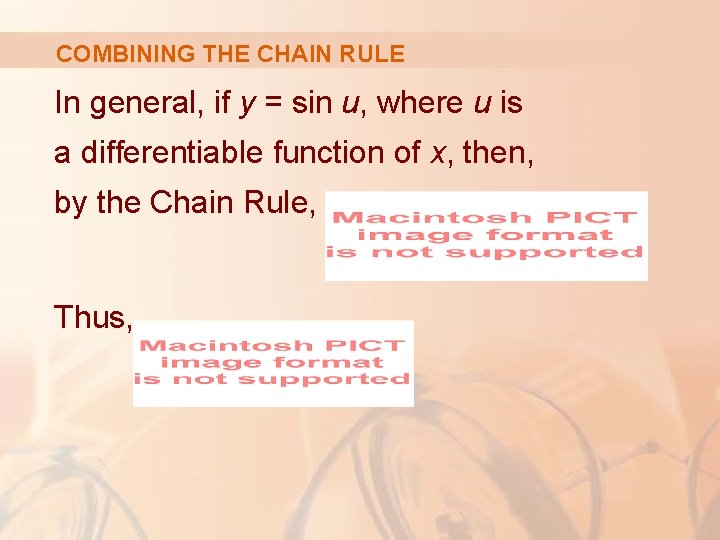COMBINING THE CHAIN RULE In general, if y = sin u, where u is a differentiable function of x, then, by the Chain Rule, Thus,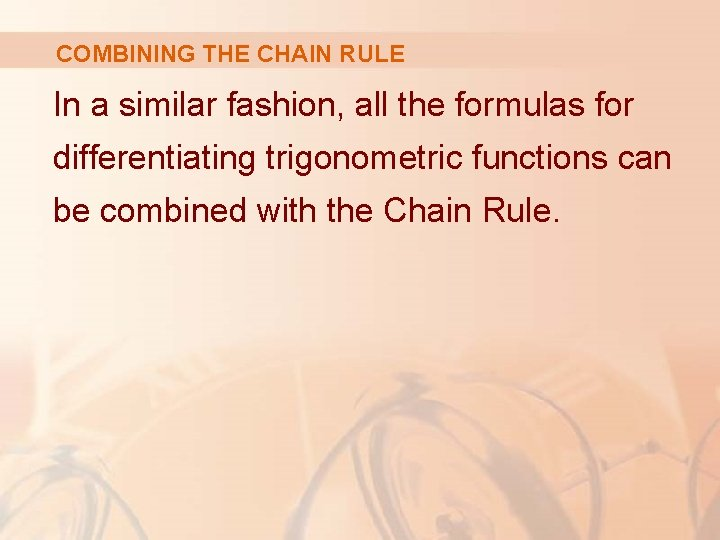COMBINING THE CHAIN RULE In a similar fashion, all the formulas for differentiating trigonometric functions can be combined with the Chain Rule.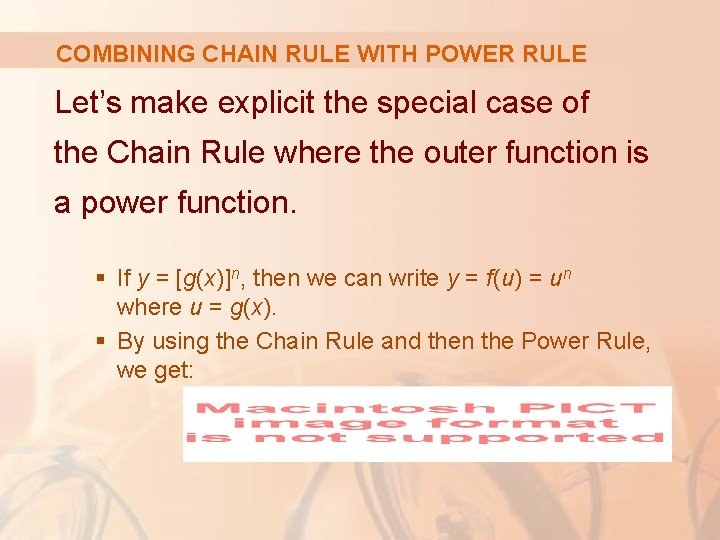COMBINING CHAIN RULE WITH POWER RULE Let’s make explicit the special case of the Chain Rule where the outer function is a power function. § If y = [g(x)]n, then we can write y = f(u) = un where u = g(x). § By using the Chain Rule and then the Power Rule, we get: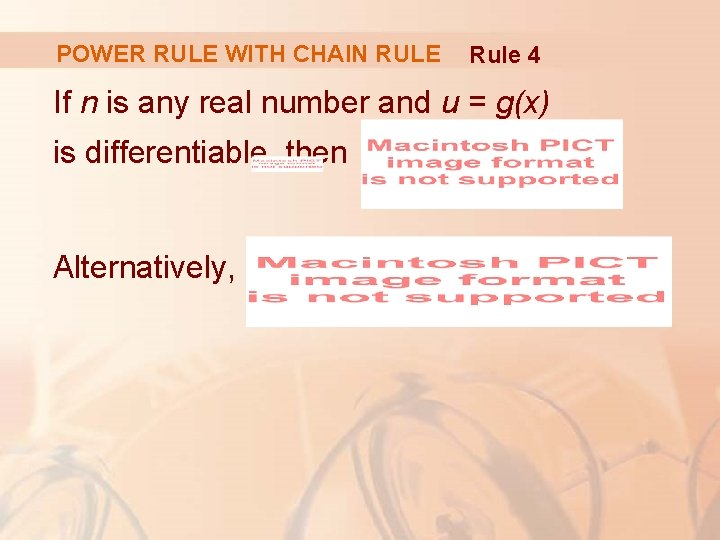POWER RULE WITH CHAIN RULE Rule 4 If n is any real number and u = g(x) is differentiable, then Alternatively,POWER RULE WITH CHAIN RULE Notice that the derivative in Example 1 could be calculated by taking n = ½ in Rule 4.POWER RULE WITH CHAIN RULE Example 3 Differentiate y = (x 3 – 1)100 § Taking u = g(x) = x 3 – 1 and n = 100 in the rule, we have:POWER RULE WITH CHAIN RULE Example 4 Find f’ (x) if § First, rewrite f as f(x) = (x 2 + x + 1)-1/3 § Thus,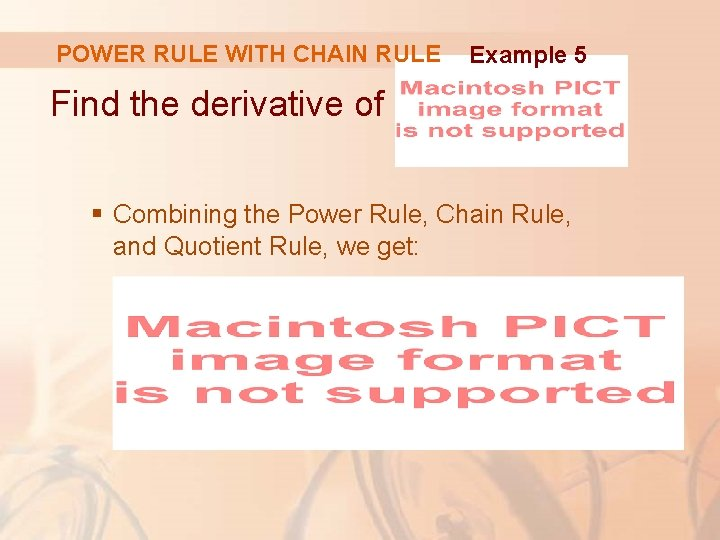POWER RULE WITH CHAIN RULE Example 5 Find the derivative of § Combining the Power Rule, Chain Rule, and Quotient Rule, we get: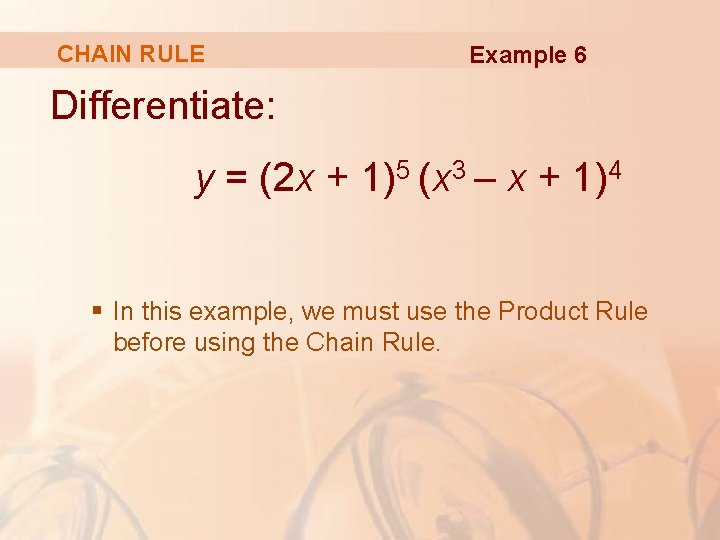CHAIN RULE Example 6 Differentiate: y = (2 x + 1)5 (x 3 – x + 1)4 § In this example, we must use the Product Rule before using the Chain Rule.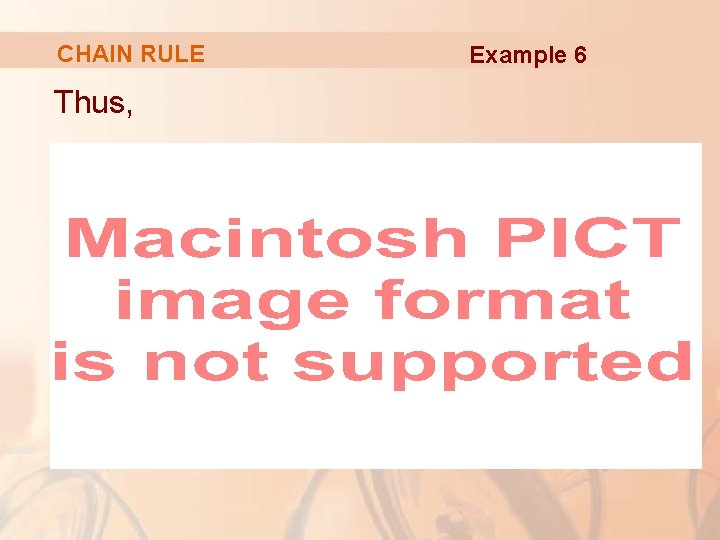CHAIN RULE Thus, Example 6CHAIN RULE Example 6 Noticing that each term has the common factor 2(2 x + 1)4(x 3 – x + 1)3, we could factor it out and write the answer as:CHAIN RULE Example 7 Differentiate y = esin x § Here, the inner function is g(x) = sin x and the outer function is the exponential function f(x) = ex. § So, by the Chain Rule:CHAIN RULE We can use the Chain Rule to differentiate an exponential function with any base a > 0. § Recall from Section 1. 6 that a = eln a. § So, ax = (eln a)x = e(ln a)x.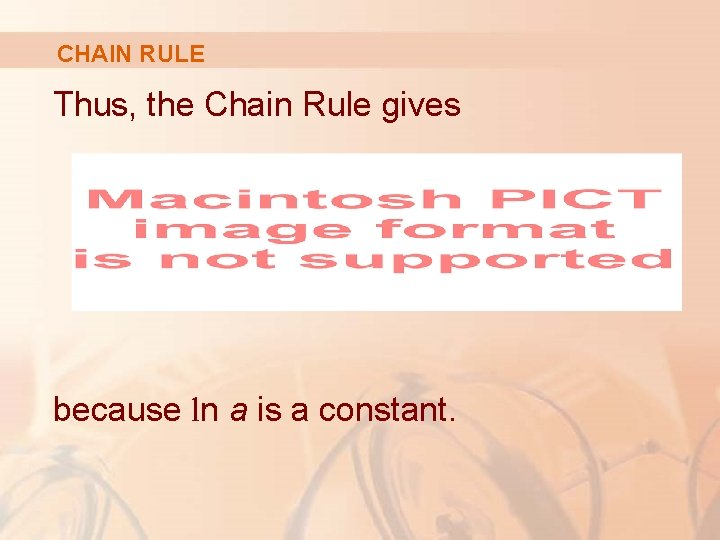CHAIN RULE Thus, the Chain Rule gives because ln a is a constant.CHAIN RULE Formula 5 Therefore, we have the formula: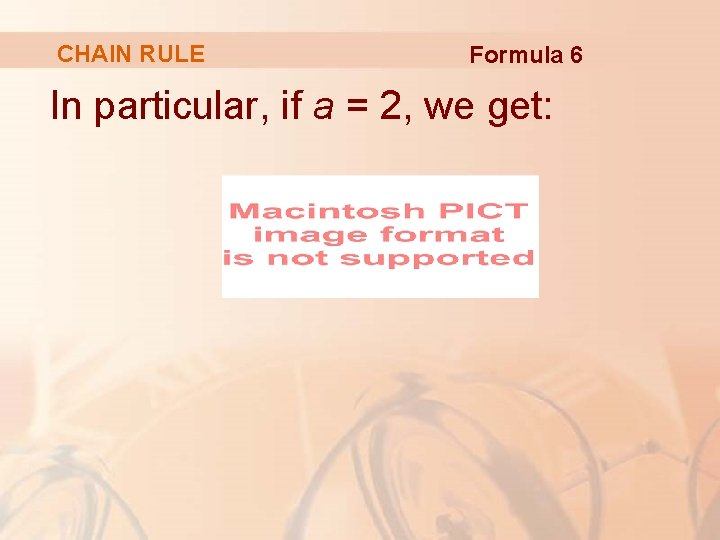CHAIN RULE Formula 6 In particular, if a = 2, we get: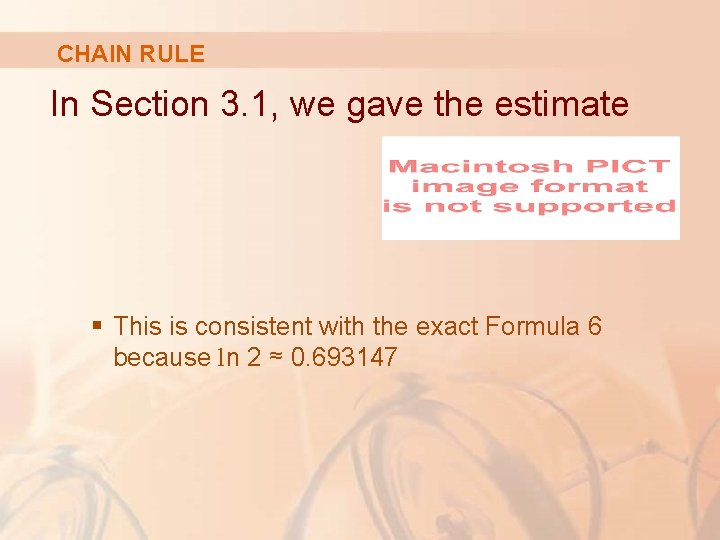CHAIN RULE In Section 3. 1, we gave the estimate § This is consistent with the exact Formula 6 because ln 2 ≈ 0. 693147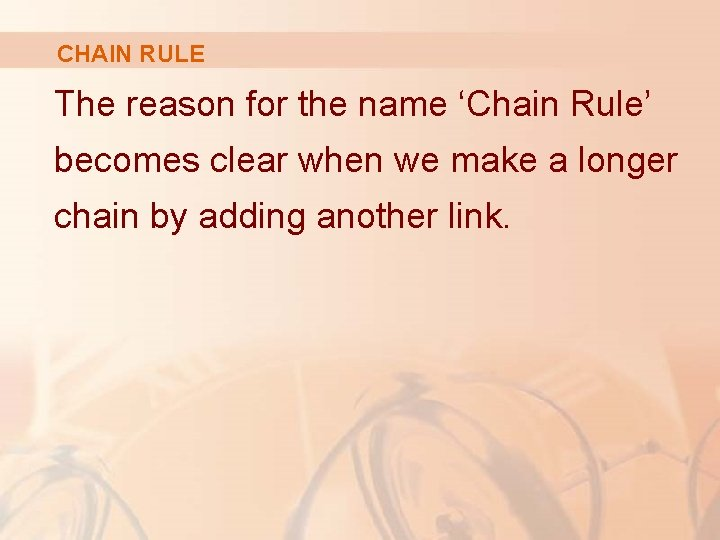CHAIN RULE The reason for the name ‘Chain Rule’ becomes clear when we make a longer chain by adding another link.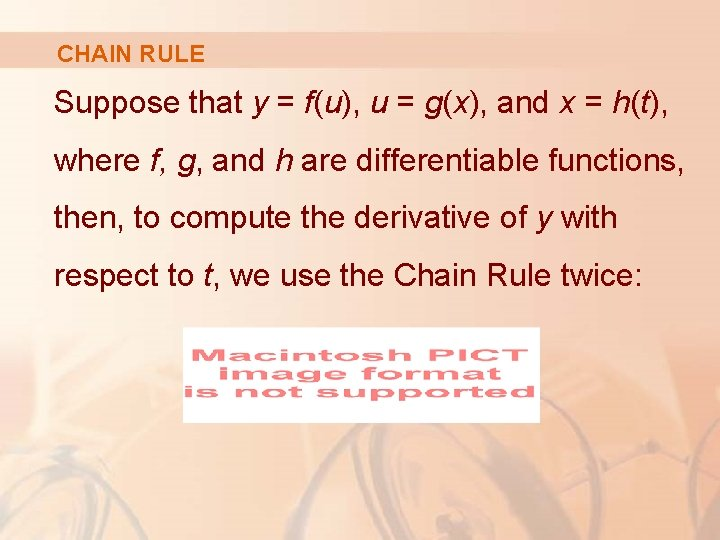CHAIN RULE Suppose that y = f(u), u = g(x), and x = h(t), where f, g, and h are differentiable functions, then, to compute the derivative of y with respect to t, we use the Chain Rule twice:CHAIN RULE Example 8 If § Notice that we used the Chain Rule twice.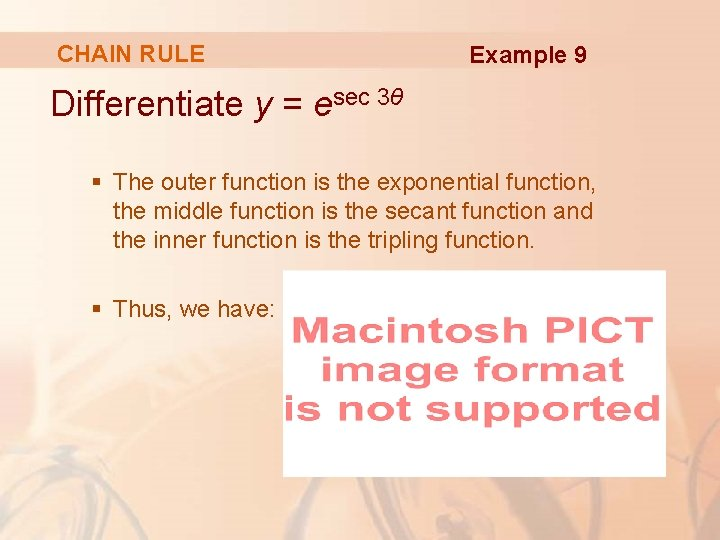CHAIN RULE Example 9 Differentiate y = esec 3θ § The outer function is the exponential function, the middle function is the secant function and the inner function is the tripling function. § Thus, we have: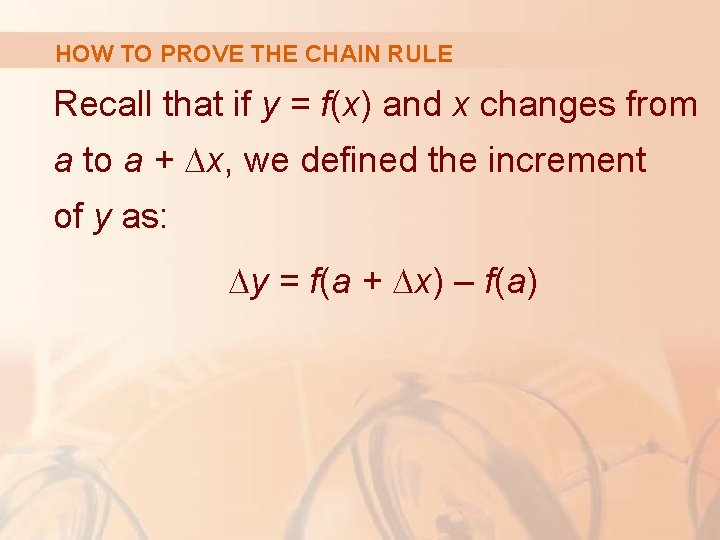HOW TO PROVE THE CHAIN RULE Recall that if y = f(x) and x changes from a to a + ∆x, we defined the increment of y as: ∆y = f(a + ∆x) – f(a)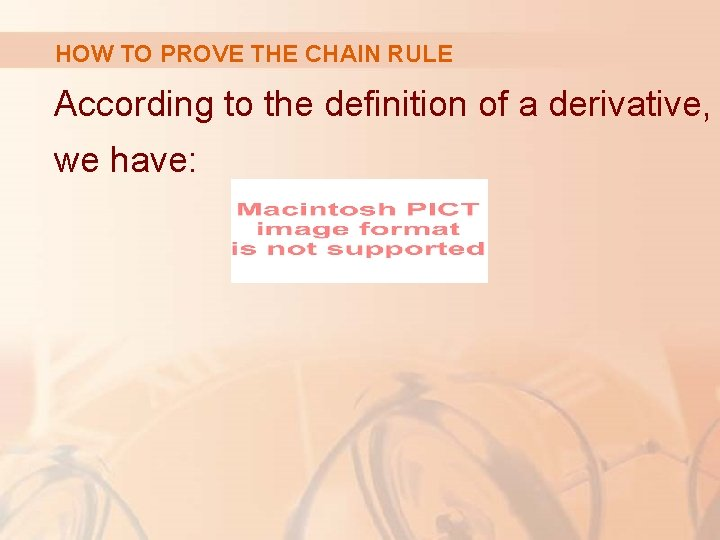HOW TO PROVE THE CHAIN RULE According to the definition of a derivative, we have: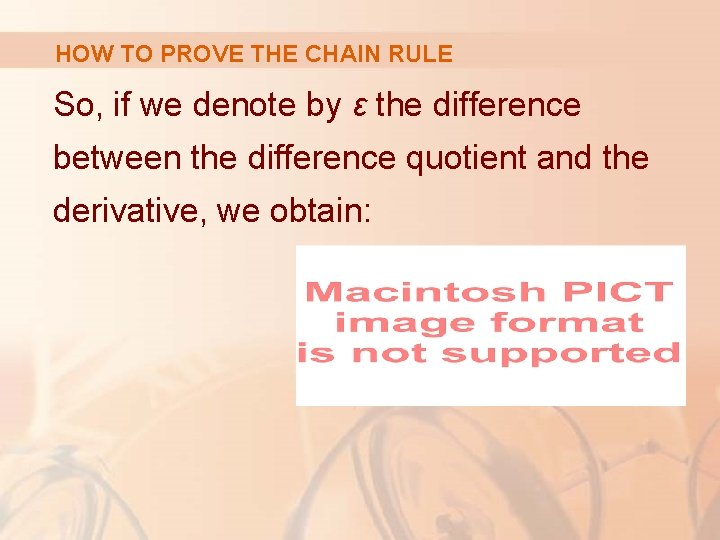HOW TO PROVE THE CHAIN RULE So, if we denote by ε the difference between the difference quotient and the derivative, we obtain:HOW TO PROVE THE CHAIN RULE However, § If we define ε to be 0 when ∆x = 0, then ε becomes a continuous function of ∆x.HOW TO PROVE THE CHAIN RULE Equation 7 Thus, for a differentiable function f, we can write: § ε is a continuous function of ∆x. § This property of differentiable functions is what enables us to prove the Chain Rule.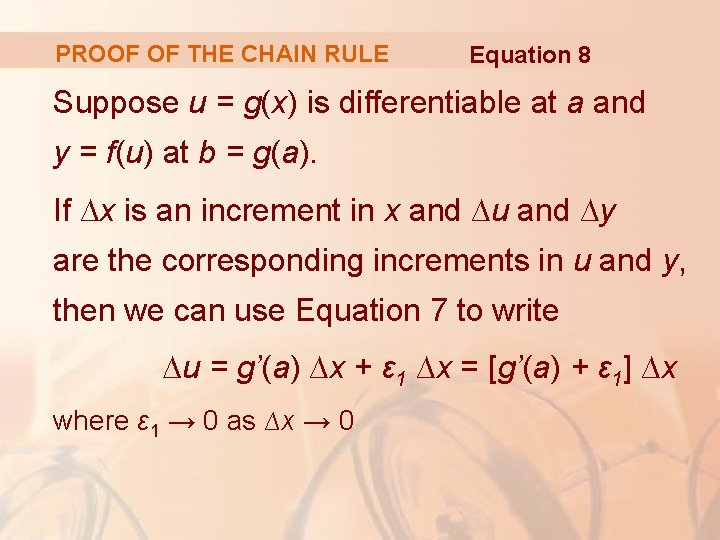PROOF OF THE CHAIN RULE Equation 8 Suppose u = g(x) is differentiable at a and y = f(u) at b = g(a). If ∆x is an increment in x and ∆u and ∆y are the corresponding increments in u and y, then we can use Equation 7 to write ∆u = g’(a) ∆x + ε 1 ∆x = [g’(a) + ε 1] ∆x where ε 1 → 0 as ∆x → 0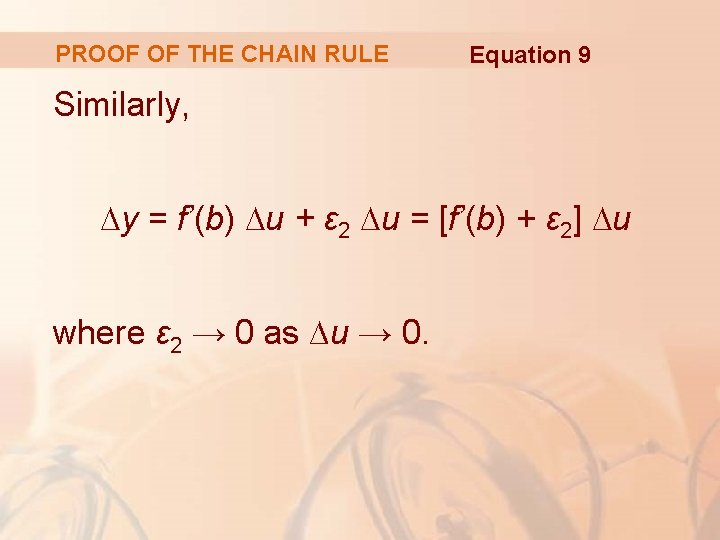PROOF OF THE CHAIN RULE Equation 9 Similarly, ∆y = f’(b) ∆u + ε 2 ∆u = [f’(b) + ε 2] ∆u where ε 2 → 0 as ∆u → 0.PROOF OF THE CHAIN RULE If we now substitute the expression for ∆u from Equation 8 into Equation 9, we get: So,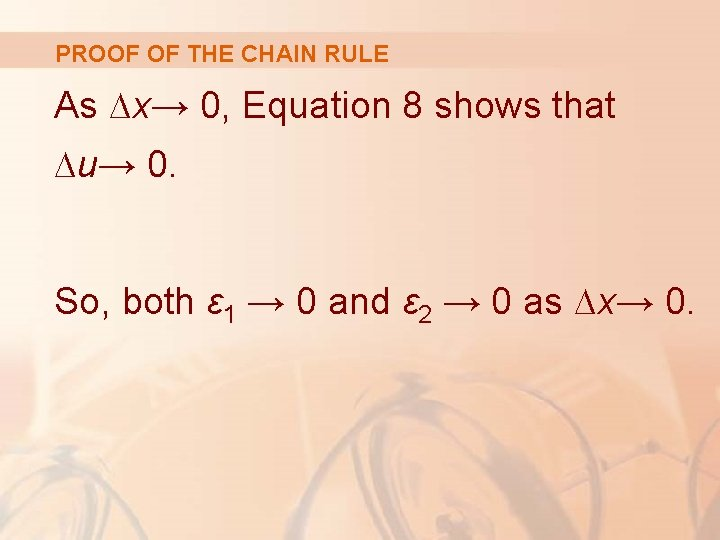PROOF OF THE CHAIN RULE As ∆x→ 0, Equation 8 shows that ∆u→ 0. So, both ε 1 → 0 and ε 2 → 0 as ∆x→ 0.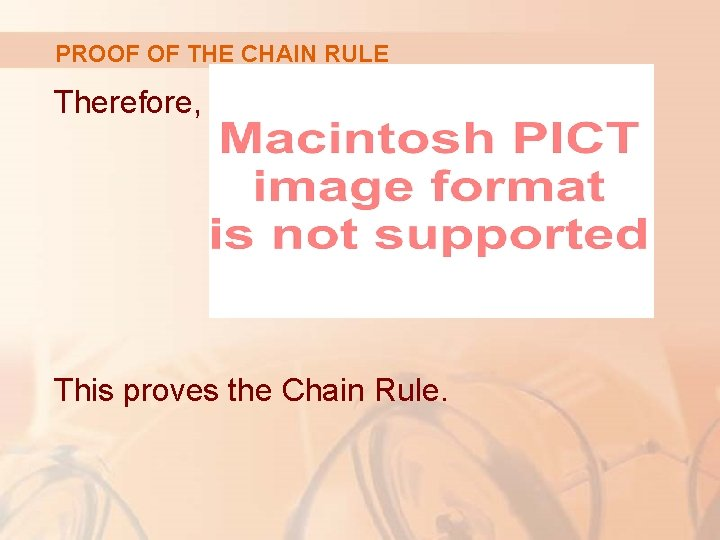PROOF OF THE CHAIN RULE Therefore, This proves the Chain Rule.